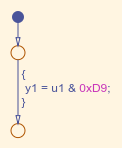# Bitwise Operations

This example shows how to implement bitwise operations by using Simulink blocks, Stateflow Charts, and MATLAB Function blocks.

To include a logical operation in your model, use the Bitwise Operator block from the Logic and Bit Operations library.

1. Open example model `ex_bit_logic_SL`.The Logical Operator blocks perform an AND operation in the model. To change the operation, double-click the block and set the Operator field to any of the operations in the menu.

2. Double-click the block to open the Block Parameters dialog box.

3. To perform bitwise operations with a bit-mask, select Use bit mask.

If another input uses bitwise operations, clear the Use bit mask parameter and enter the number of input ports.

4. In the Bit Mask field, enter a decimal number. Use `bin2dec` or `hex2dec` to convert the input from binary or hexadecimal. In this example, enter `hex2dec('D9')`.

5. To build the model and generate code, press Ctrl+B.

The code implementing the bitwise operator AND is in the `ex_bit_logic_SL_step` function in `ex_bit_logic_SL.c`:

```/* Exported block signals */ uint8_T u1; /* '<Root>/u1' */ uint8_T y1; /* '<Root>/Bitwise Operator' */ /* Model step function */ void ex_bit_logic_SL_step(void) { /* S-Function (sfix_bitop): '<Root>/Bitwise Operator' incorporates: * Inport: '<Root>/u1' */ y1 = (uint8_T)(u1 & 217); } ```

### Stateflow Chart

1. Open example model `ex_bit_logic_SF`.2. Right-click the Stateflow chart to open the chart Properties.

3. Verify that the Enable C-bit operations check box is selected. For more information, see Enable C-bit operations (Stateflow).

4. To build the model and generate code, press Ctrl+B.

The code implementing the bitwise operator AND is in the `ex_bit_logic_SF_step` function in `ex_bit_logic_SF.c`:

```/* Exported block signals */ uint8_T u1; /* '<Root>/u1' */ uint8_T y1; /* '<Root>/Bit_Logic' */ /* Model step function */ void ex_bit_logic_SF_step(void) { /* Chart: '<Root>/Bit_Logic' incorporates: * Inport: '<Root>/u1' */ y1 = (uint8_T)(u1 & 0xD9); } ```

### MATLAB Function Block

In this example, to show the MATLAB Function block method for implementing bitwise logic into the generated code, use the bitwise `OR`, '|'.

1. Open example model `ex_bit_logic_ML`.2. The MATLAB Function Block contains this function:

```function y1 = fcn(u1, u2) %#eml y1 = bitor(u1, u2); end ```

3. To build the model and generate code, press Ctrl+B.

The code implementing the bitwise operator OR is in the `ex_bit_logic_ML_step` function in `ex_bit_logic_ML.c`:

```/* Exported block signals */ uint8_T u1; /* '<Root>/u1' */ uint8_T u2; /* '<Root>/u2' */ uint8_T y1; /* '<Root>/Bitwise OR' */ /* Model step function */ void ex_bit_logic_ML_step(void) { /* MATLAB Function: '<Root>/Bitwise OR' incorporates: * Inport: '<Root>/u1' * Inport: '<Root>/u2' */ y1 = (uint8_T)(u1 | u2); } ```